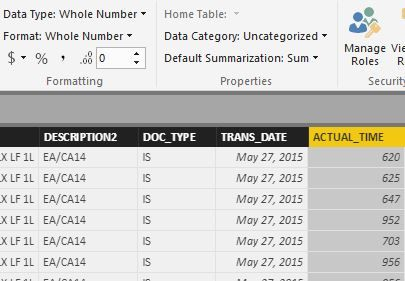cancel
Showing results for
Did you mean:Helper II

## Convert 24 HR time

Hi,

I have a column of time data in 24 Hr time:

Time

625

844

1241

1556

Where 625 is 06:25 AM.

How can I convert this into a usable form for PowerBI?

Thank you,

1 ACCEPTED SOLUTIONSuper User

The first formula I supplied is DAX. That is the one that starts with "Column =". You use that version after you have run your query and are out of the Query Editor window and in Power BI Desktop. You go to the Data tab (middle icon on the left) and go to "Modeling" and then "New Column". That is where you would use the FIRST formula.

The SECOND formula is when you are in the Query Editor window and go to "Add Column" in the ribbon and then New Column. You seem to be using the DAX query when you should be using the M query and using the M query when you should be using the DAX query.

Become an expert!: Enterprise DNA
External Tools: MSHGQM
Latest book!:
Mastering Power BI 2nd EditionDAX is easy, CALCULATE makes DAX hard...
14 REPLIES 14New Member

Maybe you want to use something shorter:

Col = REPLACE([Hora],IF(LEN([Hora])=3,2,3),0,":")New Member

Maybe you want to use something like this:

Col = REPLACE([Hora],IF(LEN([Hora])=3,2,3),0,":")Super User
`Column = CONCATENATE(CONCATENATE(LEFT([Time],LEN([Time])-2),":"),RIGHT([Time],2))`

Become an expert!: Enterprise DNA
External Tools: MSHGQM
Latest book!:
Mastering Power BI 2nd EditionDAX is easy, CALCULATE makes DAX hard...Helper II

I received the following message: Expression error: The name 'CONCATENATE' wasn't recognized. Make sure it's spelled correctly.Super User

M code version:

`= Text.Middle(Text.From([Time]),0,Text.Length(Text.From([Time])) - 2) & ":" & Text.Middle(Text.From([Time]),Text.Length(Text.From([Time]))-2,2)`

This assumes that your original data is a Number, hence the Text.From functions. If you have converted it to Text, you don't need those although the formula as presented will work with either Text or Number as the original data type for [Time] column.

Become an expert!: Enterprise DNA
External Tools: MSHGQM
Latest book!:
Mastering Power BI 2nd EditionDAX is easy, CALCULATE makes DAX hard...Helper II

The syntax for '.' is incorrect. (DAX(Text.Middle(Text.From([ACTUAL_TIME]),0,Text.Length(Text.From([ACTUAL_TIME])) - 2) & ":" & Text.Middle(Text.From([ACTUAL_TIME]),Text.Length(Text.From([ACTUAL_TIME]))-2,2))).Helper II

Is it still M or DAX?Super User

The first formula I supplied is DAX. That is the one that starts with "Column =". You use that version after you have run your query and are out of the Query Editor window and in Power BI Desktop. You go to the Data tab (middle icon on the left) and go to "Modeling" and then "New Column". That is where you would use the FIRST formula.

The SECOND formula is when you are in the Query Editor window and go to "Add Column" in the ribbon and then New Column. You seem to be using the DAX query when you should be using the M query and using the M query when you should be using the DAX query.

Become an expert!: Enterprise DNA
External Tools: MSHGQM
Latest book!:
Mastering Power BI 2nd EditionDAX is easy, CALCULATE makes DAX hard...Helper II

For the first I receive the following error:

An argument of function 'LEFT' has the wrong data type or has an invalid value.

What's the difference beteen DAX and M?Super User

What is the data type of your "Time" column? Is your Time column called "Time"? I tested with the Time column being Whole Number and Text.The difference between DAX and M are that they are two completely different languages. DAX is used with PowerPivot (the in-memory data model that Power BI uses). M is used with Power Query, the extract, transform and load (ETL) system that Power BI uses.

Become an expert!: Enterprise DNA
External Tools: MSHGQM
Latest book!:
Mastering Power BI 2nd EditionDAX is easy, CALCULATE makes DAX hard...Helper II

Whole Number for both.Super User

Try switch [ACTUAL_TIME] to Text, it is the ?Data Type" drop down. Weird. If that doesn't work, copy and paste your formula into a response so that I can copy and paste it exactly and recreate this.

Become an expert!: Enterprise DNA
External Tools: MSHGQM
Latest book!:
Mastering Power BI 2nd EditionDAX is easy, CALCULATE makes DAX hard...Helper II

I'll worry about it later. You're second query worked so I'll just work off that. Thank you!Super User

That would be because you were trying to enter a DAX formula into the Query Editor, which uses "M". Import your data and then in the Data tab, go to Modeling in the ribbon and "New Column". I'll see if I can post the equivalent M formula, but please note that you are posting to a forum on DAX Tips and Tricks, not Power Query "M" Tips and Tricks.

Become an expert!: Enterprise DNA
External Tools: MSHGQM
Latest book!:
Mastering Power BI 2nd EditionDAX is easy, CALCULATE makes DAX hard...